JEE  >  Physics Test 11 - Gravitation, Oscillation And Waves

# Physics Test 11 - Gravitation, Oscillation And Waves

Test Description

## 30 Questions MCQ Test JEE Main & Advanced Mock Test Series | Physics Test 11 - Gravitation, Oscillation And Waves

Physics Test 11 - Gravitation, Oscillation And Waves for JEE 2023 is part of JEE Main & Advanced Mock Test Series preparation. The Physics Test 11 - Gravitation, Oscillation And Waves questions and answers have been prepared according to the JEE exam syllabus.The Physics Test 11 - Gravitation, Oscillation And Waves MCQs are made for JEE 2023 Exam. Find important definitions, questions, notes, meanings, examples, exercises, MCQs and online tests for Physics Test 11 - Gravitation, Oscillation And Waves below.
Solutions of Physics Test 11 - Gravitation, Oscillation And Waves questions in English are available as part of our JEE Main & Advanced Mock Test Series for JEE & Physics Test 11 - Gravitation, Oscillation And Waves solutions in Hindi for JEE Main & Advanced Mock Test Series course. Download more important topics, notes, lectures and mock test series for JEE Exam by signing up for free. Attempt Physics Test 11 - Gravitation, Oscillation And Waves | 30 questions in 60 minutes | Mock test for JEE preparation | Free important questions MCQ to study JEE Main & Advanced Mock Test Series for JEE Exam | Download free PDF with solutions
 1 Crore+ students have signed up on EduRev. Have you?
Physics Test 11 - Gravitation, Oscillation And Waves - Question 1

### The acceleration of moon with respect to earth is 0.0027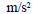and the acceleration of an apple falling on earth’s surface is about 10.Assume that the radius of the moon is one fourth of the earth’s radius. If the moon is stopped for an instant and then released, it will move towards the earth. The initial acceleration of the moon towards the earth will be

Physics Test 11 - Gravitation, Oscillation And Waves - Question 2

### The acceleration of the moon just before it strikes the earth in the previous question is

Physics Test 11 - Gravitation, Oscillation And Waves - Question 3

### A person brings a mass of 1 kg from infinity to a point A. Initially the mass was at rest but it moves at a speed of 2m/s as it reaches A. The work done by the person on the mass is –3J. The potential at A is

Physics Test 11 - Gravitation, Oscillation And Waves - Question 4
A body is suspended from a spring balance kept in a satellite. The reading of the balance is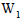when the satellite goes in on orbit of radius R and is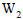when it goes in an orbit of radius 2R.
Detailed Solution for Physics Test 11 - Gravitation, Oscillation And Waves - Question 4
In either cases of different radii of R and 2R, the satellite behaves like a freely falling body. So, the acceleration in either cases = acceleration due to gravity (g). The reading of the spring balance is given by the expression W = m g Hence, the acceleration due to gravity remains the same for both and the reading of the spring balance remains same as well, in both the cases.

Physics Test 11 - Gravitation, Oscillation And Waves - Question 5

A particle is kept at rest at a distance R(earth’s radius) above the earth’s surface. The minimum speed with which it should be projected so that it does not return is

Detailed Solution for Physics Test 11 - Gravitation, Oscillation And Waves - Question 5

Using conservation of energy,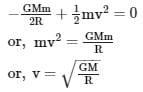Physics Test 11 - Gravitation, Oscillation And Waves - Question 6
A uniform spherical shell gradually shrinks maintaining its shape. The gravitational poential at the centre.
Detailed Solution for Physics Test 11 - Gravitation, Oscillation And Waves - Question 6 AS it is maintaining its shape so its mass will remain constant which implies that if it shrinks then its volume decreases and to keep the mass constant ,its density increases.Also the gravitational potential at the centre of a uniform spherical shell is inversely proportional to the radius of the shell with a negative sign. When a uniform spherical shell gradually shrinks, the gravitational potential at the centre decreases (because of the negative sign in the formula of potential).
Physics Test 11 - Gravitation, Oscillation And Waves - Question 7
A particle executes simple harmonic motion with a frequency v. The frequency with which the kinetic
energy oscillates is
Detailed Solution for Physics Test 11 - Gravitation, Oscillation And Waves - Question 7 As SHM is represented by
x=Asin (wt)
v=Awcos(wt)
so kE is=1/2mv^2
so kE is proportional to [cos(wt)]^2
If the time period of particle is T
Time period of x=T
Time period of kE=T/2 because:
[(cos)/(sin) function have time period 2π if their power is odd else their time period is π if power is even]
in KE the time period will be reduced to T/2 because the cos is in square while in x the sin has power one i.e the time period will be T
so v=1/T
v of KE=2/T=2v
Physics Test 11 - Gravitation, Oscillation And Waves - Question 8

A particle executes simple harmonic motion under the restoring force provided by a spring. The time period is T. If the spring is divided in two equal parts and one part is used to continue the simple harmonic motion , the time period will

Detailed Solution for Physics Test 11 - Gravitation, Oscillation And Waves - Question 8

Time period T =2pi under root l/gIf the length is halved then Time period t=2πunder root l/2gSo t=T/under root 2

Physics Test 11 - Gravitation, Oscillation And Waves - Question 9
The free end of a simple pendulum is attached to the ceiling of a box. The box is taken to a height and the point, the box is released to fall freely. As seen from the box during this period, the bob will
Detailed Solution for Physics Test 11 - Gravitation, Oscillation And Waves - Question 9 At the bottom of the pendulum, the velocity is tangential and the string with no gravity the string will restrain it forcing it to travel in a circular path.
Physics Test 11 - Gravitation, Oscillation And Waves - Question 10

Suppose a tunnel is dug along a diameter of the earth.A particle is dropped from a point, a distance h directly above the tunnel. The motion of the particle as seen from the earth is

Physics Test 11 - Gravitation, Oscillation And Waves - Question 11
A particle moves on the x-axis according to the equation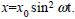The motion is simple harmonic
Physics Test 11 - Gravitation, Oscillation And Waves - Question 12
A sine wave is travilling in a medium. A particular particle has zero displacement at a certain instant. The particle closest to it having zero displacement is at a distance
Physics Test 11 - Gravitation, Oscillation And Waves - Question 13

Two waves represented by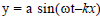and y =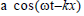are superposed. The resultant wave will have an amplitude

Physics Test 11 - Gravitation, Oscillation And Waves - Question 14

Consider two waves passing through the same string.Principle of superposition for displacement says that the net displacement of a particle on the string is sum of the displacements proudced by the two waves individually. Suppose we state similar principles for the net velocity of the particle and the kinetic energy of the particle. Such a principle will be valid for

Physics Test 11 - Gravitation, Oscillation And Waves - Question 15

Two sine waves travel in the same direction in a medium. The amplitude of each wave is A and the phase difference between the two waves is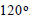The resultant amplitude will be

Physics Test 11 - Gravitation, Oscillation And Waves - Question 16
A standing wave is produced on a string clamped at one end and free at the other. The length of the string
Physics Test 11 - Gravitation, Oscillation And Waves - Question 17
Consider the following statements about sound passing through a gas
A. The pressure of the gas at a point oscillates in time
B. The position of a small layer of the gas oscilates in time
Physics Test 11 - Gravitation, Oscillation And Waves - Question 18

A tuning fork sends sound waves in air. If the temperature of the air increases, which of the following parameters will change ?

Physics Test 11 - Gravitation, Oscillation And Waves - Question 19

A cylindrical tube, open at both ends, has a fundamental frequency v. The tube is dipped vertically in water so that half of its length is inside the water. The new fundamental frequency is

Physics Test 11 - Gravitation, Oscillation And Waves - Question 20
A tuning fork of frequency 512 Hz is vibrated with a sonometer wire and 6 beats per second aer heard. The beat frequency reduces if the tension in the string is slightly increased. The original frequency of vibration of the string is
Physics Test 11 - Gravitation, Oscillation And Waves - Question 21

A small source of sound moves on a circle as shown in the figure and an observer is standing on O. Let n1,n2 and n3 be the frequencies heard when the source is at A, B and C respectively. Then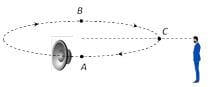Detailed Solution for Physics Test 11 - Gravitation, Oscillation And Waves - Question 21

At point A, source is moving away from observer so apparent frequency n1<n (actual frequency) At point B source is coming towards observer so apparent frequency n2<n and point C source is moving perpendicular to observer so n3 =n Hence n2>n3>n1

Physics Test 11 - Gravitation, Oscillation And Waves - Question 22

A mass m is placed inside a hollow sphere of mass M as shown in figure. The gravitational force on mass m is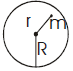Physics Test 11 - Gravitation, Oscillation And Waves - Question 23
A point P (R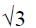,0,0) lies on the axis of a ring of mass M and radius R. The ring is located in y-z plane with its centre at origin O. A small particle of mass m starts from P and reaches O under gravitational attraction only. Its speed at O will be
Physics Test 11 - Gravitation, Oscillation And Waves - Question 24

In the figure, the block of mass m, attached to the springnof stiffness k is in contact with the compeletly elastic wall, and the compression in the spring is e. The spring is compressed further by e by displacing the block towards left and is, then released. If the collision between the block and the wall is completely elastic, then the time period of oscillations of the block will be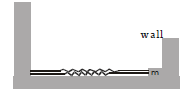Physics Test 11 - Gravitation, Oscillation And Waves - Question 25

A wire of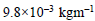passes over a frinctionless light pulley fixed on the top of frinctionless inclined plane which makes an angle of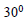with the horizontal.Masses m and M are tied at the two ends of wire such that m rests on the plane and M hangs freely vertically downwards. The entire system is in equilibrium and a transverse wave propagates along the wire with a velocity of 100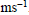Choose the correct option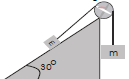Physics Test 11 - Gravitation, Oscillation And Waves - Question 26
Direction : Read the following question and choose
A. If both Assetion and Reason are correct and reason is the correct exaplanation of assertion
B. If both Assertion and Reason are true,but Reason is not correct explanation of the Assertion
C. If Assertion is true, but the Reason is false
D. If Assertion is false, but the Reason is true
E. If both assertion and reason are false
Assertion : Compression and rarefaction involve changes in density and pressure
Reason : When particles are compressed, density of medium increases and when they are rerefied, density of medium decrease
Physics Test 11 - Gravitation, Oscillation And Waves - Question 27
Direction : Read the following question and choose
A. If both Assetion and Reason are correct and reason is the correct exaplanation of assertion
B. If both Assertion and Reason are true,but Reason is not correct explanation of the Assertion
C. If Assertion is true, but the Reason is false
D. If Assertion is false, but the Reason is true
E. If both assertion and reason are false
Assertion : On a rainy day sound travel slower than on a dry day
Reason : When moisture is present in air the density of air increases
Physics Test 11 - Gravitation, Oscillation And Waves - Question 28
Direction : Read the following question and choose
A. If both Assetion and Reason are correct and reason is the correct exaplanation of assertion
B. If both Assertion and Reason are true,but Reason is not correct explanation of the Assertion
C. If Assertion is true, but the Reason is false
D. If Assertion is false, but the Reason is true
E. If both assertion and reason are false
Assertion : If oil of density higher than that of water is used in place of water in a resonance tube, the frequency decreases
Reason : Frequency does not depend on change of medium in resonance tube
Physics Test 11 - Gravitation, Oscillation And Waves - Question 29
Direction : Read the following question and choose
A. If both Assetion and Reason are correct and reason is the correct exaplanation of assertion
B. If both Assertion and Reason are true,but Reason is not correct explanation of the Assertion
C. If Assertion is true, but the Reason is false
D. If Assertion is false, but the Reason is true
E. If both assertion and reason are false
Assertion : In simple harmonic total mechanical energy can be negative also
Reason : Potential energy is always negative and if it is greater than kinetic energy total mechanical
energy will be negative
Physics Test 11 - Gravitation, Oscillation And Waves - Question 30
Direction : Read the following question and choose
A. If both Assetion and Reason are correct and reason is the correct exaplanation of assertion
B. If both Assertion and Reason are true,but Reason is not correct explanation of the Assertion
C. If Assertion is true, but the Reason is false
D. If Assertion is false, but the Reason is true
E. If both assertion and reason are false
Assertion : In SHM let x be the maximum speed, y the frquency of oscilation and z the maximum
acceleration then xy /z is a constant quanity
Reason : The is becasue xy /z becomes a dimensionless quantiy

## JEE Main & Advanced Mock Test Series

2 videos|325 docs|189 tests
 Use Code STAYHOME200 and get INR 200 additional OFF Use Coupon Code
Information about Physics Test 11 - Gravitation, Oscillation And Waves Page
In this test you can find the Exam questions for Physics Test 11 - Gravitation, Oscillation And Waves solved & explained in the simplest way possible. Besides giving Questions and answers for Physics Test 11 - Gravitation, Oscillation And Waves, EduRev gives you an ample number of Online tests for practice

## JEE Main & Advanced Mock Test Series

2 videos|325 docs|189 tests